# What’s the Path of Your Data?

## Introducing Path Charts (and how to create them!)

In my last post, I challenged bar charts by introducing the snail chart. Though being more of a fun example, it made me think about how I could dig deeper and create an alternative for the other most common graph type in town, the line chart.

We all know them. Line charts are the traditional choice for displaying trends in datasets, with a (time) interval on the x-axis and some continuous data on the y-axis. They appear in yearly reports showing revenue changes in a company. They show predictions for the global temperature change to help us understand what challenges humankind faces.

But what happens if you want to get a feeling about a trend of ordinal data? Let’s take a look at this based on a sample case.

# Sample Case: Amazon Review Data

Let’s assume you are an author who published the rather nonfamous book How to Avoid Huge Ships: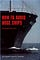“How to Avoid Huge Ships” — by Captain John W. Trimmer

Over ten years have passed since publishing this book, and once again the time of year arrived where you take the routine check of your sales statistics on Amazon. And to your great surprise, you noticed that all of a sudden your sales went through the roof recently. Now you really want to find out why! So you are checking all possibilities:

• Are there more huge ships to avoid these days?
• Is climate change evolving faster than you thought and everybody needs boats now?
• Or does it have something to do with the reviews on Amazon?

After extensive research, you figured out that it may have something to do with those reviews. So you write a script (well, you are a writer, right?) to extract the review data of your book. But it is all numbers … You need to visualize it!

Now, you could go with a line chart, the most common way of showing a trend, to find any anomalies in recent times. But … Nah! … You are creative! Line charts are for anybody else! So let’s see what else is out there:

Before starting, you want to get some inspiration. So you ask Google to show some unique variations of line charts:

“Mmm, not really.”

“That even doesn’t make any sense for my data … next, please!”

“OK, ~~ slope, you say?”

“I think we are getting somewhere here!”

“Hello, sugar!”

That piece by Nadieh Bremer encodes the decimal digits of pi in a specific angle and, thus, creates something like a path (“Mh … I may call this kind of chart exactly that: Path Chart”). It was actually based on a chart which is already over 100 years old, created by John Venn (yes, the same guy who also invented the Venn Diagram):

When compared to the traditional line chart, similarities still exist:

• It consists of edges and nodes
• It displays a sequence

But the difference lays, for one, in the representation of the data instance:

Line Chart:

Edges in a line chart do not carry any info about the data instance but rather serve as a connection between those. Here the node carries the info of the data through its position in the 2D space and the line is only an additional cue to understand the trend better.

Path Chart:

In a path chart, the edge is the data instance as it carries the info of the data with its angle as a cue and the node serves as the connection between two lines.

You could argue that a line chart can be plotted without the lines as it is nothing else than an ordered scatterplot.

Another difference is that there are no real axes in path charts but rather a focal point that serves as a starting position from where the line emerges into the 2D space. The term path chart is also best explained by visualizing this emergence:The emergence of the path for the decimal digits of pi using the distribution from “The Art of Pi”

When seeing this animation, it may remind you of a street network, where each intersection (=node) is a decision point from where the next street (=path) should be taken to reach your destination (=represent your data).

OK, now that you know what the path chart is, you want to apply it to your data!

Let’s try to create a path chart for your data using the d3.js library.

First, you include the SVG settings and load the data:

`<div id="mainDiv"></div><script>//------------------////--CREATE THE SVG--////------------------//const svg = d3.select("#mainDiv").append("svg")const g = svg.append("g")//-----------------////--LOAD THE DATA--////-----------------//d3.csv("rating.csv").then(function(data){//Here the magic happens})</script>`

Inside your function, you are going to create the path chart by creating SVG `<line>` tags for each rating instance. Let’s first enter these lines into our d3 workflow:

`//-----------------////--LOAD THE DATA--////-----------------//d3.csv("ratings.csv").then(function(d){  //----------------------------// //--ENTER THE PATHs AS LINES--// //----------------------------//  let chartLine = g.selectAll('.thePath')  .data(data)   let newLine = chartLine  .enter()  .append("line")   .style("stroke-width", 2)   .style("stroke-linejoin", "round")   .style("stroke-linecap", "round")`

Now that you entered the lines, you need to understand how you actually want to draw the path. Basically, the ending coordinates of one line should become the starting coordinates of its successor line. Let’s create a small function that computes this for you:

`const getCoords = () => {    const lastLine = d3.selectAll('.thePath').filter((d,i,list) => i === list.length - 1)                const x = parseFloat(lastLine.attr("x2"))    const y = parseFloat(lastLine.attr("y2"))     return {         "x": x,         "y": y         }}`

Now you can continue to the update step, where you need to set initial parameters such as the line length, the angle of the line as well as its coordinates. After this, you loop through the lines, calculate their angle based on the rating value, followed by the assignment of the coordinates and their update using your `getCoords()` function (and because you knew you had ten ratings before their sudden rise you are going to draw these 10 rating lines in red):

`//----------// //--UPDATE--// //----------// //initialize the parameters of the lines const lineLength = 18 const leftMargin = 100 const topMargin = 200  let angle, endCoordX, endCoordY = 0 let coords = {"x": leftMargin, "y": topMargin}//number of rating categories const ratingCats = 5//loop through the lines and create their geometry   newLine.each(function(d,i) {  //calculate the angle position  angle = (2*Math.Pi) / ratingCats * d.rating;//calculate the end coordinates of the line  endCoordX = lineLength*Math.cos(angle) + coords["x"]   endCoordY = lineLength*Math.sin(angle) + coords["y"]//draw the line (highlight first 10 ratings)  d3.select(this)   .attr("class", "thePath")   .style("stroke", ()=> (i<10) ? "#f00" : "#000")   .attr('x1', coords["x"])   .attr('y1', coords["y"])   .attr('x2', endCoordX)   .attr('y2', endCoordY)//update initial coordinates for next line  coords = getCoords() })`

Finally, you add a basic legend and adjust the dimension of the SVG container:

`//add legend g.append("rect")     .attr("x", 10)     .attr("y", 10)     .attr("width", 155)     .attr("height", 90)     .style("stroke", "#999")     .style("fill", "#fff")     g.append('text')     .attr("x", 20)     .attr("y", 30)     .style("font-size", "15px")     .style("fill", "#444")     .style("font-weight", 800)     .style("font-family", "Arial")     .text("Rating Encoding")const rootCoord = {"x": 80, "y": 70}for (var i=1; i<=ratingCats; i++) {     angle = (2 * Math.PI) / ratingCats * i;     let xCoord = lineLength*Math.cos(angle) + rootCoord["x"]     let yCoord = lineLength*Math.sin(angle) + rootCoord["y"]             g.append("line")       .style("stroke", "#000")       .style("stroke-width", 1.2)       .attr('x1', rootCoord["x"])       .attr('y1', rootCoord["y"])       .attr('x2', xCoord)       .attr('y2', yCoord)          g.append('text')         .attr("x", xCoord - 5)         .attr("y", yCoord + 5)         .style("font-size", "15px")         .style("fill", "#444")         .style("font-weight", 800)         .style("stroke", "#fff")         .style("stroke-width", 0.5)         .style("font-family", "Arial")         .text(i) }  svg     .attr("width", g.node().getBBox().width + leftMargin)     .attr("height", g.node().getBBox().height + topMargin)`

Et voila, your first path chart:

Nice! So, what does this tell you now? Something is definitely still odd here. The chart is not really intuitive. The reason might be the 360° angle distribution, which causes lines to intersect each other (go back to “The Art of Pi” visualization, in which this issue creates a little mess). Also, recognize the 1-star rating is a direct neighbor of the full 5-star rating? This means that a path with an eastward trend just like yours could be both highly-rated or poorly-rated. What you want to achieve is making these two ratings being furthest away from each other. When talking about angles, it would mean you want them to be opposite angles. So let’s adjust our `angle` calculation inside our loop and make the path follow an eastward global direction:

`angle = ((Math.PI / (ratingCats-1)) * (ratingCats - d.rating)) - (0.5 * Math.PI);`

This would lead to the following path chart:

OK, so the trend is clear: UP! This means your book seems to have received a lot of high ratings. But there is still an issue here: Thinking about your opposite angle solution again, you realize that if a 1-star rating were followed by a 5-star rating (or vice versa), their lines in the path chart would overlap each other. So they should not be precisely opposite:

`angle = (Math.PI * (ratingCats - d.rating — 2)) / ratingCats`

Now you have a much more readable solution:

This is the final path representation of the rating data. Just a last annotation to display the average value:

A path chart turns out to be actually useful. You can see that your average rating previous to the boom was not really bad, but also not as good as it is now. This is due to a high amount of 5-star ratings that you received recently, many of them in succession. This means: Let’s take a look at the reviews!

# Use Cases & Caveats

Similar to many other chart types, a path chart may be helpful for only a very narrow subset of use cases. As pointed out earlier, the path chart probably makes the most sense for ordinal data with a limited amount of categories or a continuous variable that has a (more or less) predefined range. Its strength lays in the simultaneous visualization of an overall trend, its average, and each single data instance.

However, there is one big issue that I personally could not really solve yet (and that may have no satisfactory solution). In an earlier draft of this article, I was about to post the following image of the chart that I thought would be much more readable and helpful because of the additional gridlines and axis: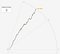Do you see that the true average of 4.25 looks more like to be located at 4.5 on the arc axis?

But then I found a mathematical flaw in my assumptions about the path chart. Here is a visual explanation for this problem: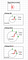The problem of adding an axis in a path chart. Red lines are the lines for the rating values, the black line the true average line, and the circle represents the axis with the green numbers being the tick marks for the rating value. (Note: In this example, I took the opposite angle convention.)

In the first two cases on top, you can see that the black line with the angle representing the average of a rating of 4 stars exactly intersects with the end node of the path. However, in the third case on the bottom, the path’s end node location is not at the average line (3.25 stars). This means that the end node does not represent the overall average’s angle and thus, an axis and gridline cannot be included. The reason for this problem is due to weight differences of a single instance in the overall dataset. If there is a trend going one direction and suddenly an opposite value enters the path, its weight is higher than that of the previous lines, thus the distance to the average line increases

After some trial and errors, I found a workaround that does preserve the correct average value, but at the cost of a decreasing line length as the path grows. In this solution, I adjust the line length of the newest line by extending or shrinking it to its intersection point with the current average line. The resulting graph would look like this: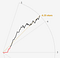A possible solution by adjusting single line lengths. (Note: To make it easier to see the decreasing line length, I marked each line’s end node.)

Here you can judge the whole trend by looking. For instance, you can see now that the first ten reviews were constantly under a 4-star rating and only since then the trend went over this border. But with an increasing number of data instances, the influence of a single line gets progressively lower, which means its length decreases as well. Hence, the advantage of judging individual data points is lost in the above solution.

If you think you may know another trick to include a gridline/axis of some kind, I would be pleased to hear it!

As we know now, with an increasing number of data, the path will become too large to analyze both the overall trend and each data instance. Additionally, the chart most certainly will extend the viewport. For this, some tweaks can be implemented to keep the path within sight (such as decreasing the single line length or the angle difference between categories). It also may be helpful to keep the average of the trend as the horizontal baseline, thus, rotating the chart.

Furthermore, a path chart cannot project total amounts of each category. If for your rating example, the overall path is located at a 3-star rating, it does not mean that most ratings had actually 3 stars. It even does not mean that there is any 3-star rating at all in the dataset.

A final disadvantage is the lack of an x-axis. It is impossible to know at which date a rating happened by looking at your final solution (the first ten ratings were 10 years apart whereas the last 90 were made within two months). Thus it may be helpful to add annotations to the chart to see crucial points in the sequence of the lines.

If you are interested in an implementation of the path chart you can head over to my DataViz project One Line, One Community (?), where I analyzed data of the Data Visualization Society 2019 Survey and drew the whole article with a single continuous line using path charts to show some of the survey’s results (even though it may not be a trend analysis the path chart matched perfectly with my single line convention).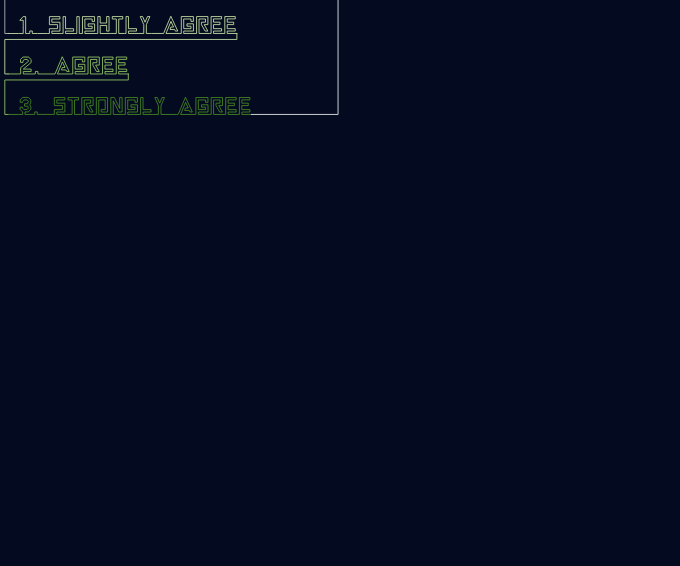My “One Line, One Community (?)” DataViz project, using path charts to visualize Likert scale data

Finally, I would be happy to know what you think about the path chart. Is it something you would use in your project? Do you find it intuitive, or do you have a hard time reading it? Let me know!

In the same series:

## Nightingale

### By Nightingale

Keep up with the latest from Nightingale, the journal of the Data Visualization Society Take a look

Written by

Written by

## Joseph (Geolic)

#### A freelance DataViz expert and GIS engineer. Life Goal: Riding bicycle from Berlin (Germany) to Busan (Korea).